## Table of ContentOpen Access

ARTICLE

# A New Intrusion Detection Algorithm AE-3WD for Industrial Control Network

Yongzhong Li1,2,*, Cong Li1, Yuheng Li3, Shipeng Zhang2

1 School of Computer Science and Engineering, Taizhou Institute of Sci. and Tec. NJUST, Taizhou, 225300, China
2 School of Computer Science, Jiangsu University of Science and Technology, Zhenjiang, 212003, China
3 Suzhou Institute of Technology, Jiangsu University of Science and Technology, Suzhou, 215600, China

* Corresponding Author: Yongzhong Li. Email:Journal of New Media 2022, 4(4), 205-217. https://doi.org/10.32604/jnm.2022.034778

## Abstract

In this paper, we propose a intrusion detection algorithm based on auto-encoder and three-way decisions (AE-3WD) for industrial control networks, aiming at the security problem of industrial control network. The ideology of deep learning is similar to the idea of intrusion detection. Deep learning is a kind of intelligent algorithm and has the ability of automatically learning. It uses self-learning to enhance the experience and dynamic classification capabilities. We use deep learning to improve the intrusion detection rate and reduce the false alarm rate through learning, a denoising AutoEncoder and three-way decisions intrusion detection method AE-3WD is proposed to improve intrusion detection accuracy. In the processing, deep learning AutoEncoder is used to extract the features of high-dimensional data by combining the coefficient penalty and reconstruction loss function of the encode layer during the training mode. A multi-feature space can be constructed by multiple feature extractions from AutoEncoder, and then a decision for intrusion behavior or normal behavior is made by three-way decisions. NSL-KDD data sets are used to the experiments. The experiment results prove that our proposed method can extract meaningful features and effectively improve the performance of intrusion detection.

## Keywords

1  Introduction

Intrusion detection systems are important to prevent security threats and protecting networks from attacks. In recent year, Industrial Control Network security is the hot topic of network security. Industrial control network systems are widely used in many enterprises that are the lifeblood of national economy, as petroleum, electric power, transportation, water conservancy. Information security of Industrial control network is related to Internet network security and social stability. Industrial control networks are interacting with Internet and external network more and more closely with the continuous progress of integration of normalization and industrialization. The traditional industrial control network that considered as closed and isolated now are broken, and the security problems of Internet and external network are also introduced to industrial control network system more severe.

Stuxnet Virus  sounded the alarm of industrial control network security in 2010, the attacks against industrial control systems have occurred frequently. In October 2014, the Korean nuclear power plant virus, the December 2015 Ukrainian grid power outage, and the March 2016 German nuclear power plant, In 2018, Venezuela’s national power grid was attacked. A series of security incidents such as viruses indicate that the networked development of industrial control systems has led to an increase in system security risks and intrusion threats, and the network security problems  are also more prominent. At present, industrial control system intrusion detection technologies  are still in the initial stage, the security technology of industrial control network is not perfect. At the present stage, the threats of attacks against industrial control systems are organized, large-scale, highly concealed, and lasting for a long time. Due to industrial control systems use of industry specific communication protocols, operating systems, hardware and software, but there is no corresponding security defense measures for industrial control network, making the system insecurity. Vulnerabilities of industrial control network are easily exploited by attackers for destructive operations from the perspective of external network environments. Because of industrial networks use TCP/IP technology for communication, from Internet IT system attacks can easily enter the industrial control systems, making industrial control systems more secure challenge.

The deep learning concept was proposed in 2006 by Hinton et al. (Hinton, GE, Osindero, S, & Teh, YW, 2006; Hinton, GE, & Salakhutdinov, RR, 2006). The deep learning theory is a multi-level deep learning model by imitating human thinking patterns. Machine learning is one of the main research field of artificial intelligence. Machine learning uses a variety of intelligent algorithms to enable machines to learn the potential rules from a large amount of identification data and use these rules as a basis to identify and classify new samples. Deep learning has a perfect ability to solve complex problems. So we introduce deep learning method to solve the problem of network intrusion detection system.

Intrusion detection of industrial control network has become a hotspot in industrial control security research field. In recent year, Professor Geoffrey Hinton  proposed deep learning with deep level, nonlinear, layer-by-layer feature extraction, which is a typical field of artificial intelligence in image processing , speech recognition natural language processing and other aspects  have been promoted. The essence is to build more hidden layer machine learning models and use a large amount of training data to train, get more useful data features, and finally achieve classification and efficient classification accuracy. In this paper, we proposed an improved auto-encoder and three-way decisions intrusion detection method (AE-3WD), Due to the characteristics of high completeness in deep learning to high-dimensional data feature extraction , so we use unsupervised deep learning to extract data features, and then use the three-way decisions to label the extracted features for supervised training classification, the experiments prove that AE-3WD can effectively improve the intrusion detection rate, false positive rate, and is suitable for industrial control network’s intrusion detection with high stability.

2  Relate Works

2.1 AutoEncoder

AutoEncoder is a representative model in deep learning. Like traditional machine learning, deep learning can be divided into supervised learning and unsupervised learning. AutoEncoder is a common unsupervised learning method. It can be used for features extraction and data generation. The AutoEncoder reconstructs the input as possible as original data by learning the best parameters. Compared with the linear features obtained by traditional shallow feature extraction methods such as principal component analysis (PCA), the features obtained from the encoder are non-linear, and the expression ability of the features is more powerful. The AutoEncoder  is a deep learning network for learning effective coding. AutoEncoder is belong to an unsupervised learning algorithm which is improved the basis of deep neural networks. The concept of AutoEncoder is gradually considered to be a data generation model. As shown in Fig. 1.Figure 1: AutoEncoder model

The training algorithm of the AutoEncoder mainly adopts the unsupervised learning algorithm of backward propagation, the optimization goal is to make the target output as equal as possible to the model input. The AutoEncoder process can be divided into two steps of encoding and decoding, it is usually not used alone. Encoding can expressed as the encoder GE and decoding is decoder GD. The encoder output layer is usually called the hidden layer. We define WRm×n as weight matrix and vector b for the encoder parameters, and define WRn×m as weight matrix and vector b for the decoder parameters.

The encoder can be mapped into the input vector x to representation h (x map to a set of binary hidden expressions v, v (i) ∈ [0, 1]) of the encoder hidden layer with the mapping as following:

h=GE(X)=fθ(X)=s(WX+b)(1)

Here the θ = w, b, f are Encoder parameters, and s is a non-linear activation function. As an example, ReLU (Rectified Linear Unit) are the activation function. Then, objective vector h is mapped into a reconstructed vector X by the following formula:

X=GD(h)=gθ(h)=s(Wh+b)(2)

where the parameters θ=(W,b) is the decoder g. The purpose is to make WT=W. In order to reduce the number of parameters, we can minimize the reconstructed error to optimize the parameters as the following form:

argminθ,θ1Ni=1NL(xi,gθ(fθ(xi)))=argminθ,θ1Ni=1NL(xi,xi)(3)

where the L is a measure function for the encoder between input and output, and N is the number of samples. The error function is the mean square error function in our approaches, expressed as following:

L(xi,xi)=||xixi||2(4)

In the process of using AutoEncoder, we choose the denoising AutoEncoder  because of its advantages:

(1)   By adding noise in the input data can relieve the overfitting problem of AutoEncoder;

(2)   By adding noise in the input data can avoid the AutoEncoder to learn simple mapping function;

(3)   The denoising AutoEncoder can learn the robustness represent.

Therefore, we choice the denoising AutoEncoder to process the data dimensionality reduction in our approaches. The denoising AutoEncoder reconstructs the data vector X by introducing a random noise into the original data X, The denoising AutoEncoder are trained by as the way of basic AutoEncoder.

2.2 Three-way Decisions Theory

Three-way decisions theory come from rough sets theory . It is proposed by Professor Yao Yiyu who gives an overall framework for three-way decisions. There are two state sets and three action sets were be used to describe the decision process. The state set are Ω = {Χ, ¬Χ} to present the dada or events, and the action set are A = {ɑP, ɑB, ɑN} to represent the decision:Acceptance, Delayed, and Rejection for an event, respectively. Let λPP, λBP, λNP denote the loss or cost function under the three actions of ɑP, ɑB, and ɑN when x belongs to Χ. Let λPN, λBN, λNN represent the loss function of three types of actions when x does not belong to Χ when ɑP, ɑB, ɑN are taken. Therefore, the expected loss values under the three actions ɑP, ɑB and ɑN are expressed as following:

R(αP|[x])=λPPP(X|[x])+λPNP(X|[x])(5)

R(αB|[x])=λBPP(X|[x])+λBNP(X|[x])(6)

R(αN|[x])=λNPP(X|[x])+λNNP(X|[x])(7)

According to Bayesian criterion, for the action set A = {ɑP, ɑB, ɑN} with the smallest expected loss value is selected as the best decision, and we use the POS (Χ), BND (Χ), and NEG (Χ) to represent the positive domain, the boundary domain, and the negative domain, respectively. It is generally assumed that: 0≤λPP ≤λBPNP, 0≤λNN ≤λBNPN, then the conditions of the three decision criteria (POS), (BND), (NEG) are shown in Table 1: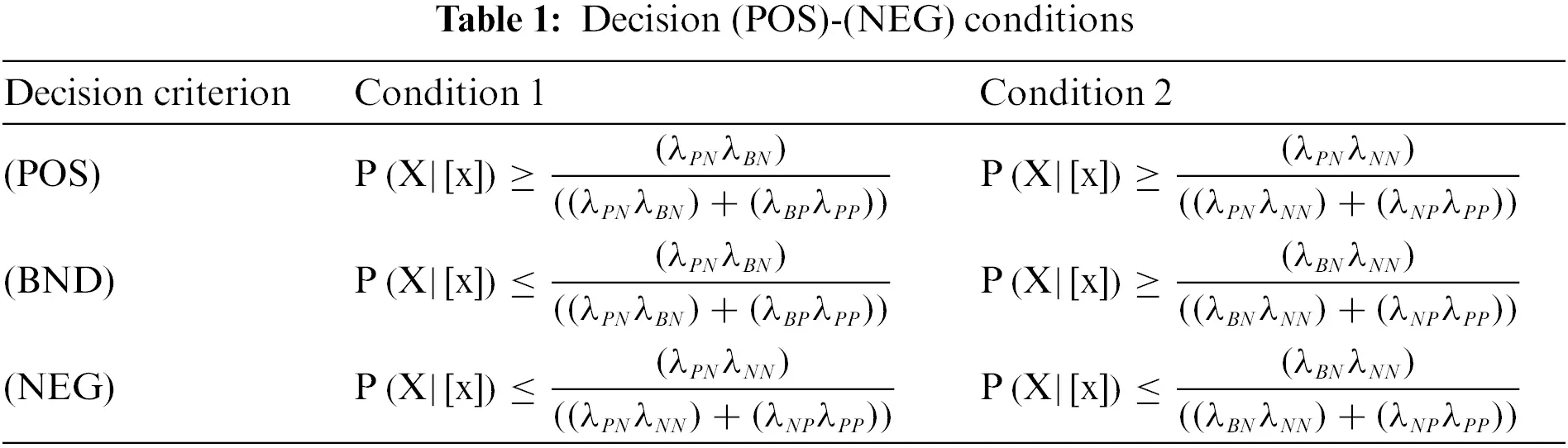For a sample or event, there may be three decisions: Acceptance or positive domain (POS), Rejection or negative domain (NEG) and Deferred decision or boundary domain (BND). There are two possible states: a positive domain (POS) that a sample belongs to positive domain or negative domain (NEG) that a sample not belong to the domain. According to three-way decisions theory, the relevant cost λ for these three possible decisions and two possible states are shown in Table 2.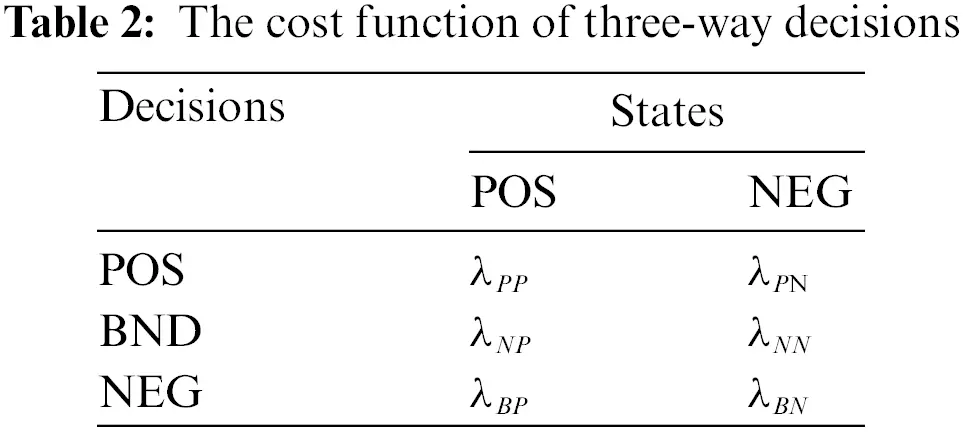The probability that a sample x belongs to a set C is P (C|x), we can define C as a positive domain, then P (Cc|x) is the probability that a sample x does not belong to domain C, the probability that x belongs to the negative domain Cc. According to the relevant theorem , that have proved that:

If P(C|x)>α, xPOS; If P(C|x)<β, xNEG; If βP(C|x)α, xBND.

Where α, β are threshold parameters that can be defined by the following formulas:

α=(λPNλBN)(λPNλBN)+(λBPλPP)(8)

β=(λBNλNN)(λBNλNN)+(λNPλPP)(9)

Since intrusion detection is a decision-making system, it is also a typical three way decision-making system. So the three-way decision theory provides a useful method for intrusion detection system.

In the process of three-way decisions, the key is to set the decision threshold {α,β}. In general, the loss function should be set according to the different analysis problems and actual situations, and according to expert experience and prior knowledge. If three-way decisions are applied to the field of intrusion detection, the selection of loss functions are impotent, they will be rooted in the field of intrusion detection. According to the intrusion detection field and our experience, the loss function set is shown in Table 3.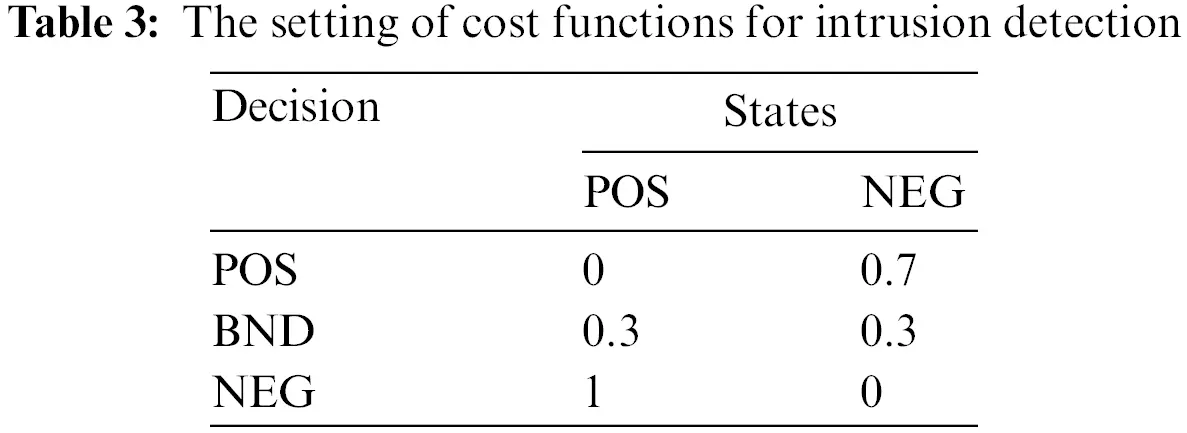The loss function has been determined, the relevant threshold {α,β} can be obtained by using the formula described above.

3  Intrusion Detection Method AE-3WD

3.1 Description of Detection Method

The intrusion detection method based on AutoEncoder and three-way decisions proposed in this paper can be divided into two parts, namely feature extraction part and intrusion detection part. The intrusion detection algorithm’s overall flowchart is shown in Fig. 2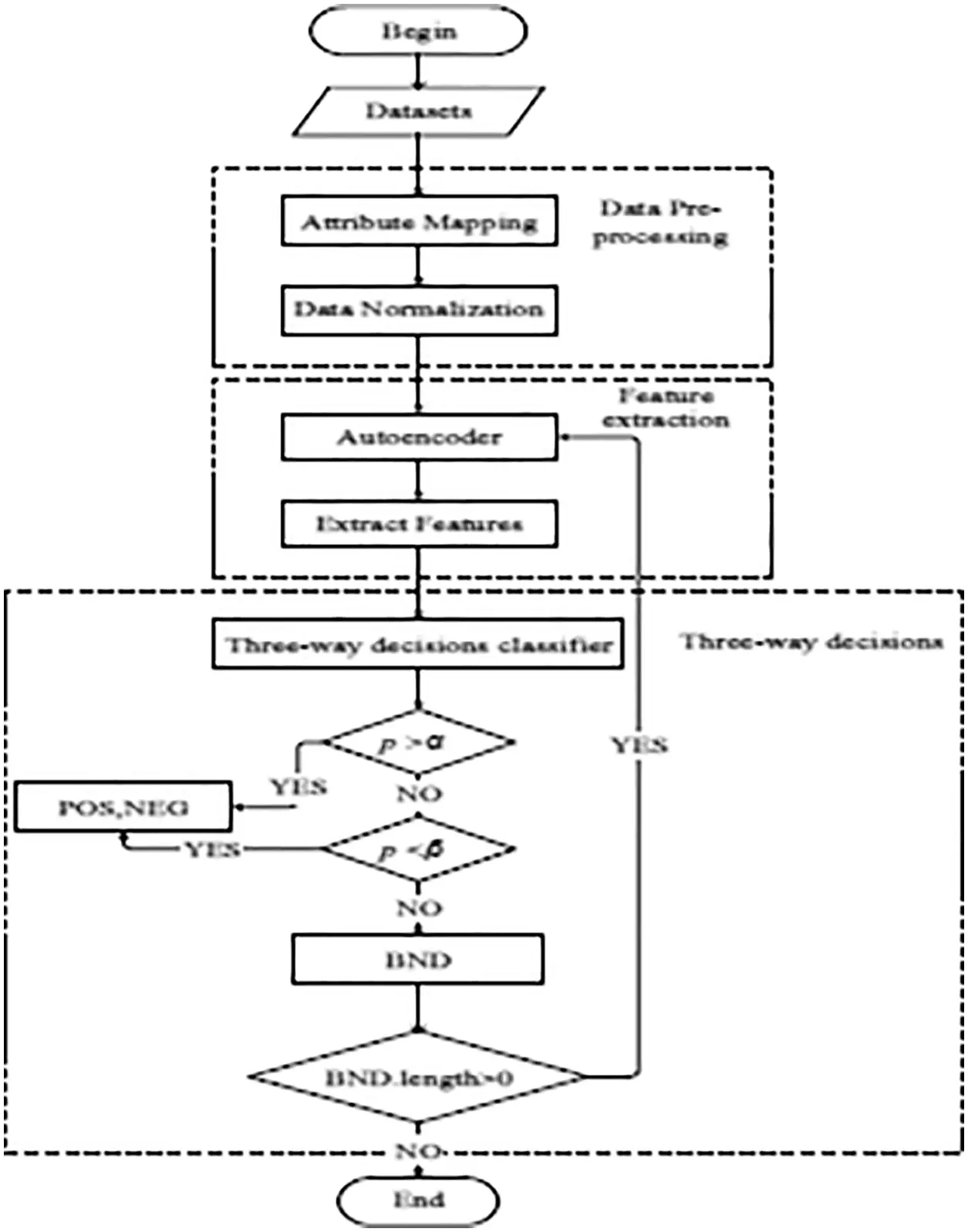Figure 2: The overall flow of intrusion detection method

As Fig. 2, the intrusion detection method AE-3WD can be divided into the three modules: (1) Data preprocessing that is to normalize the attribute data; (2) Feature extraction by using AutoEncoder; (3) Classification by using three-way decisions.

3.2 Data Preprocessing

The dataset used in intrusion detection and industrial control network intrusion detection is NSL-KDD dataset and NSL-KDD dataset. These data sets must be preprocessed before used in intrusion detection program. First, we need to convert character data into numeric data. That is to convert the character attribute of the protocol to a numeric value. For an example, the network protocol that attribute values (TCP, UDP, ICMP, character data) can be expressed as (1, 2, 3, numeric data). Secondly, it is necessary to standardize the data that to eliminate the errors that caused by different dimensions or large differences in the data. In this paper, we use Eq. (10) to normalize the attribute value that is for the range [0, 1].

x=xminimaximini(10)

In the equation, x is the i-th attribute value of column, mini is the minimum value of the i-th attribute column, and maxi is the maximum value of the i-th attribute column.

3.3 Intrusion Detection Algorithm AE-3WD

In the intrusion detection algorithm AE-3WD, we assumes that input data set are X = {x1, x2,…, xn}, the reconstructed data set are X′ = {x1′, x2′,…, xn′}. The object of the AutoEncoder is to make the result X′ as possible as to close the original input data set X, so that the original data features can be extracted by AutoEncode in the hidden layer. So AutoEncoder is to minimize the reconstructed error function to obtain optimized network parameters weights and offsets. The error function as shown in Eq. (11):

W,W,b,b=argminW,W,b,b(J(W,b))(11)

where, W and b are the weights and offsets of the Encoder, which are also network parameters required for extracting low dimensional features, W′ and b′ represent the weights and offsets of the decoder respectively.

The gradient descent method is used to update the weight parameters of the whole network, and the optimal solution of the objective function is obtained .

The algorithm AE-3WD is described as follows.

The final decision is made for the input data as samples that are belong to positive domains (POS) or negative domains (NEG). But the data in the boundary domain (BND) are need to decision again after obtaining additional information. If some samples are still divided into the boundary domain again, this decision process must be continued until all samples are divided into positive or negative domains.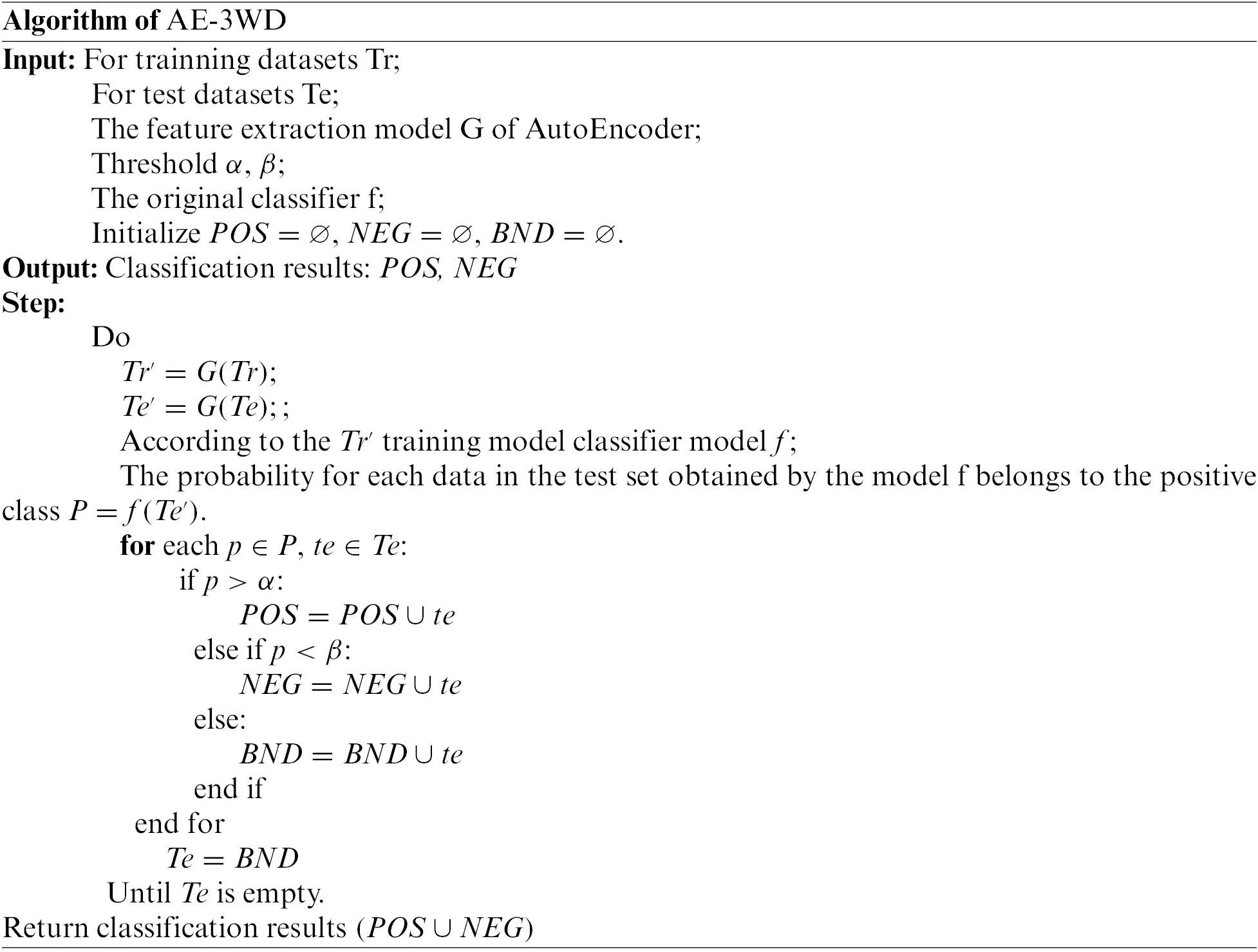4  Experimental Results and Analysis

In this section evaluates the performance of the proposed algorithm. All experiments are implemented in the PC, environment OS is Windows10, hardware is Intel (R) core (TM) i5-8250 CPU @ 1.60Ghz 1.80 GHz, 8GB DDR2-DRAM. The algorithm is implemented in Python 3.7.

4.1 Data Set Preprocessing

The datasets used to our approach experiment is the intrusion detection dataset NSL-KDD. There are 41 feature attributes and 1 class label in the NSL-KDD dataset. For different network attack behavior, the dataset NSL-KDD includes a train set and a test set for different type, as shown in Table 4.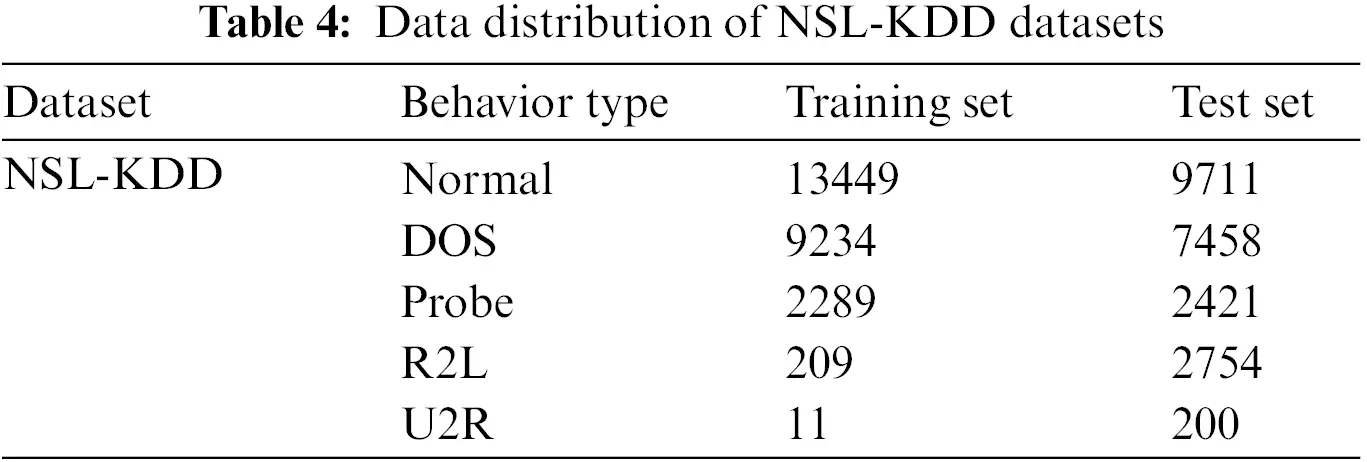In the NSL-KDD dataset, there are normal data and attack data, the attack behavior data can be divided into the four types: DoS (Denial of Service), Probe, U2R (User to Root), and R2L (Remote to Local). In the experiments, we selected 20% of the training set data and all the test data for the experiments.

4.2 Performance Evaluation

Because of the data set is uneven distribution, so it is not appropriate that only use accuracy to judge the advantages and disadvantages of the algorithm. In the intrusion detection system, there are two important evaluation indicators: False Alarm Rate and False Negatives Rate. The accuracy rate indicates how many of the network behaviors predicted to be abnormal are really abnormal behaviors; F1 score comprehensively considers the calculation results of model precision and recall, and is an important indicator to reflect the quality of the algorithm. Therefore, in our experiments, we choose five indicators that are used to judge the performance of machine learning algorithm to evaluate the intrusion detection algorithm’s performance, namely Accuracy (ACC = (TP + TN)/(TP + FP + TN + FN), Detection Rate (DR = TP/(TP + FN)), Precision (PR = TP/(TP + FP)), False Positive Rate (FPR==FP/(TN + FP)), and F1-score (F1 = 2TP/(2TP + FP + FN)). Where the TP and TN are the network attack records and normal records have been correctly classified; FP is the normal records that were mistaken to classify as attacks; FN is attack records that were mistaken to classify as normal records.

4.3 Experiment and Result Analysis

When comparing with other algorithms, in this paper we mainly consider the performance of AutoEncoder and three-way decisions. We have down two Experiment to verify the availability and effectiveness of our algorithm AE-3WD, we conducted two experiments: Experiment 1 is to verify whether AutoEncoder is better than the traditional method, and also to prove whether the classification method based on three-way decisions is better than the traditional two-way decisions approach. Experiment 2 is mainly to compare the performance of our algorithm AE-3WD with other intrusion detection algorithms. The experiments are repeated 10 times to eliminate the impact of randomness, and the mean value of each indicator are recorded.

4.3.1 Experiment 1

Experiment 1 mainly explores the impact of introducing the three-way decisions theory into the field of intrusion detection. Under the same condition of using AutoEncoder for feature extraction, it compares the performance of the three-way decisions with traditional two-way decisions classification methods in the intrusion detection system field. So we chose the PCA (Principal Component Analysis) and ICA (Independent principal Component Analysis) as the comparative reference method of AutoEncoder, their classification is same approach based on three-way decisions. The SVM (Support Vector Machine) and KNN (K-Nearest Neighbors) are used to compare with the method based on three-way decisions, and the denoising AutoEncoder is used to extract features of the input data. The results of different methods are shown in Table 5.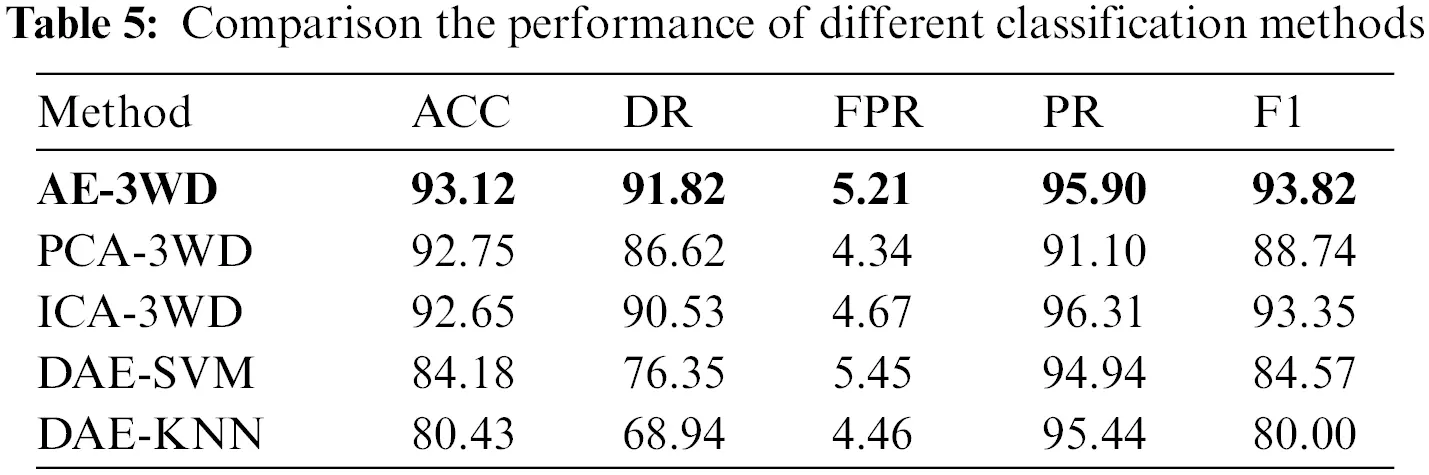From the Table 5, we can obtained the result that our method AE-3WD is better than others. The proposed AE-3WD algorithm has the higher performance in accuracy, detection rate and F1-score. Fig. 3 shows the ROC curve of algorithms.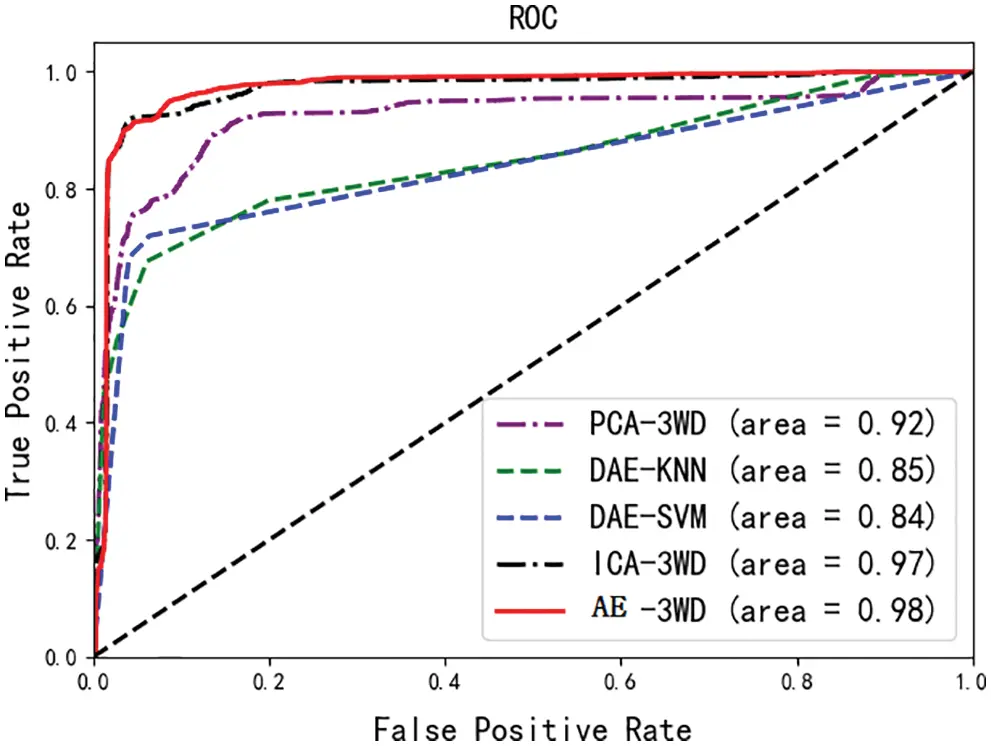Figure 3: ROC curves of different algorithms

From the Fig. 3, the ROC curve of AE-3WD is closer to (0, 1) and has the largest AUC area. It proved the effectiveness of the AE-3WD.

4.3.2 Experiment 2

Experiment 2 is mainly to compare the performance of the AE-3WD algorithm with other intrusion detection algorithms. So we choose the typical intrusion detection algorithms SFID , SNADE  and ICA-DNN  as the comparative reference algorithms. SNDAE is an intrusion detection algorithm based on stacked non-symmetric deep learning; ICA-DNN is an intrusion detection algorithm based on ICA (Independent Component Analysis)  and DNN (Deep Neural Network) . Table 6 shows the comparison between the AE-3WD and other algorithms in the same experimental environment.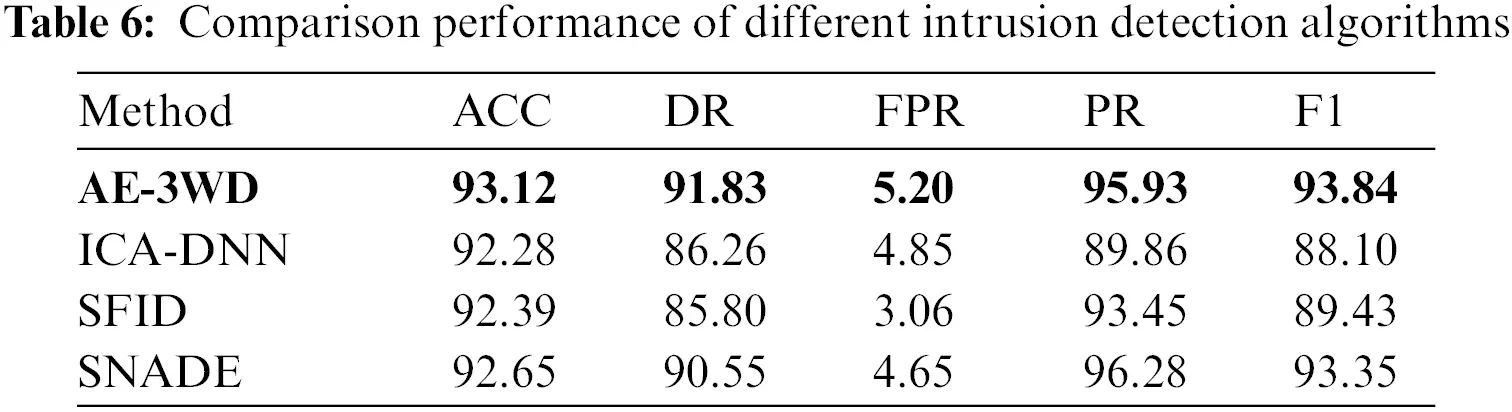The experimental results shown that AE-3WD algorithm is better than other algorithms in these indicators: Accuracy, Detection Rate and F1 score, especially in the Detection Rate. Although the AE-3WD does not perform as well as some methods in the PR, but from the overall, AE-3WD is still better than others.

The ROC curves of these algorithms are shown in the Fig. 4.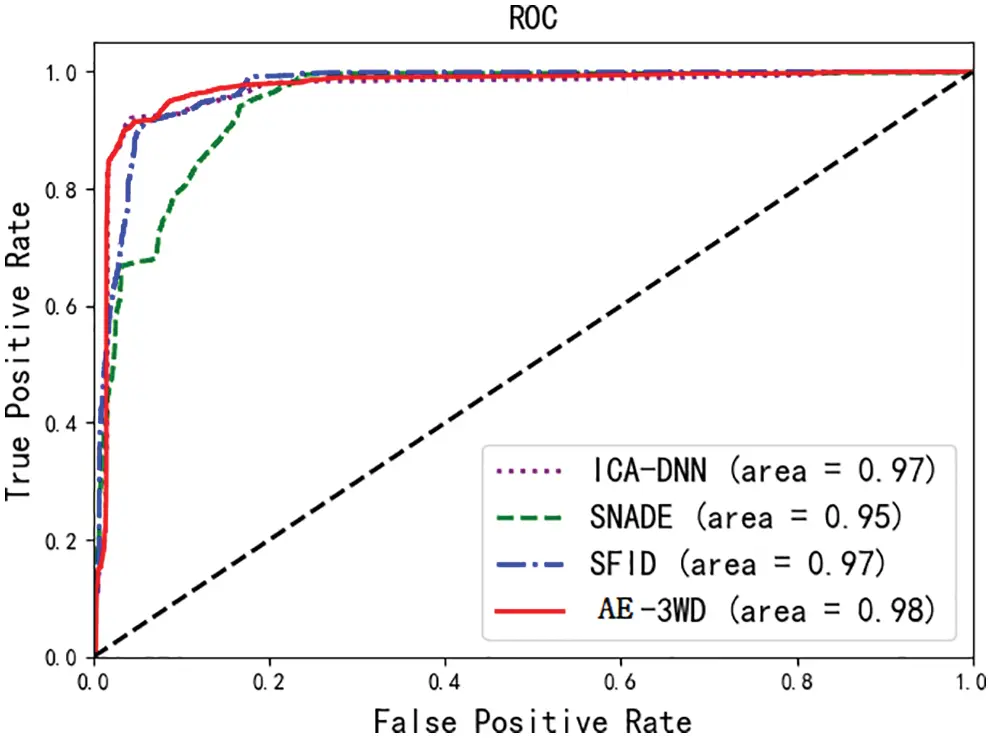Figure 4: ROC curve different approaches

From the Fig. 4, the ROC curves that AE-3WD algorithm and other algorithms are intersect somewhere, and this phenomenon proved that the performance of these algorithms are closer. But the area of the AE-3WD is larger than others, so our method is better than others.

5  Conclusion

According to the existing research, we propose a intrusion detection algorithm based on AutoEncoder and three-way decision for the security problem of industrial control network in this paper. The denoising AutoEncoder is used to extract the features from the original input data, and then the three-way decisions are used to make the classification decisions. The simulation results show that the performance of our algorithm is better than other algorithms.

In the process of classification decision-making, the three-way decision theory is used. In AE-3WD algorithm, there is not consider the time cost for repeat processing of the boundary domain when using three-way decisions theory for classification. In future research, time cost can be considered to be included in the disposal of boundary areas.

Acknowledgement: The authors would like to show their deepest gratitude to the anonymous reviewers for their constructive comments to improve the quality of the paper.

Funding Statement: Project supported by National Nature Science Foundation of China (Grant No. 61471182); Postgraduate Research & Practice Innovation Program of Jiangsu Province (Grant No. KYCX20_2993), Jiangsu postgraduate research innovation project (SJCX18_0784);

Conflicts of Interest: The authors declare that they have no conflicts of interest to report regarding the present study.

## References

1. S. Karnouskos, “Stuxnet worm impact on industrial cyber-physical system security,” in Conf. of the IEEE Industrial Electronics Society, pp. 4490–4494, 2011.
2. Colbert, E. J. M., & Hutchinson, S. (2016). Intrusion detection in industrial control systems. Cyber-Security of SCADA and Other Industrial Control Systems, 66, 209-237. [Google Scholar]
3. Papamartzivanos, D., Mármol, F. G., & Kambourakis, G. (2019). Introducing deep learning self-adaptive misuse network intrusion detection systems. IEEE Access, 7, 13546-13560. [Google Scholar]
4. Yang, Y. Q., Zheng, K. F., Wu, C. H., & Yang, Y. X. (2019). Improving the classification effectiveness of intrusion detection by using improved conditional variational AutoEncoder and deep neural network. Sensors, 19(11), 2528-2547. [Google Scholar]
5. Vinayakumar, R., Alazab, M., Soman, K. P., Poornachandran, P., & Al-Nemrat, A. (2019). Deep learning approach for intelligent intrusion detection system. IEEE Access, 7, 41525-41550. [Google Scholar]
6. Liu, W., Ci, L. L., & Liu, L. P. (2020). A new method of fuzzy support vector machine algorithm for intrusion detection. Applied Sciences, 10(3), 1065-1083. [Google Scholar]
7. Zhang, L. B., Li, H. X., Zhou, X. Z., & Huang, B. (2014). Multi-granularity cost-sensitive three-way decision for face recognition. Journal of Shandon University: Natural Science, 49(8), 48-57. [Google Scholar]
8. Bengio, Y., Courville, A., & Vincent, P. (2013). Representation learning: A review and new perspectives. IEEE Transactions on Pattern Analysis and Machine Intelligence, 35(8), 1798-1828. [Google Scholar]
9. X. Lu, Y. Tsao, S. Matsuda and C. Hori, “Speech enhancement based on deep denoising AutoEncoder,” in Proc. Interspeech, pp. 436–440, 2013.
10. Yao, Y. (2010). Three-way decisions with probabilistic rough sets. Information Sciences, 180(3), 341-353. [Google Scholar]
11. Maldonado, S., Peters, G., & Weber, R. (2020). Credit scoring using three-way decisions with probabilistic rough sets. Information Sciences, 507, 700-714. [Google Scholar]
12. Liu, D., & Decui, L. (2016). Generalized three-way decisions and special three-way decisions. Front Computer Sci Technology, 11(3), 502-510. [Google Scholar]
13. Nauman, M., Azam, N., & Yao, J. T. (2016). A Three-way decision making approach to malware analysis using probabilistic rough sets. Information Sciences, 374, 193-209. [Google Scholar]
14. Liu, Y. Y., & Du, L. N. (2015). Three-way decisions-based incremental learning method for support vector machine. Computer Science, 42(6), 82-87. [Google Scholar]
15. Liu, D., Li, T. R., & Li, H. X. (2013). Rough set theory: A three-way decisions perspective. Journal of Nanjing University: Nat. Sci. Ed, 49(5), 574-581. [Google Scholar]
16. Zhang, L., Li, H., Zhou, X., & Huang, B. (2020). Sequential three-way decision based on multi-granular AutoEncoder features. Information Sciences, 507, 630-643. [Google Scholar]
17. Feng, W. Y., Guo, X. B., & He, Y. Y. (2019). Intrusion detection model based on feedforward neural network. Netinfo Security, 9, 101-105. [Google Scholar]
18. Shone, N., Ngoc, T. N., & Phai, V. D. (2018). A deep learning approach to network intrusion detection. IEEE Transactions on Emerging Topics in Computational Intelligence, 2(1), 41-50. [Google Scholar]
19. Liu, J. H., Mao, S. P., & Fu, X. M. (2019). Intrusion detection model based on ICA algorithm and deep neural network. Netinfo Security, 19(3), 143-149. [Google Scholar]
20. Maseer, Z. K., Yusof, R., Mostafa, S. A., Bahaman, N., & Musa, O. (2021). Deepiot.ids: Hybrid deep learning for enhancing IoT network intrusion detection. Computers, Materials & Continua, 69(3), 3945-3966. [Google Scholar]
21. El-Shafai, W., El-Nabi, S. A., El-Rabaie, E. M., Ali, A. M., & Soliman, N. F. (2022). Efficient deep-learning-based AutoEncoder denoising approach for medical image diagnosis. Computers, Materials & Continua, 70(3), 6107-6125. [Google Scholar]

Y. Li, C. Li, Y. Li and S. Zhang, "A new intrusion detection algorithm ae-3wd for industrial control network," Journal of New Media, vol. 4, no.4, pp. 205–217, 2022.This work is licensed under a Creative Commons Attribution 4.0 International License , which permits unrestricted use, distribution, and reproduction in any medium, provided the original work is properly cited.

View

Like

## Related articles

• Shuren Zhou, Wenlong Liang, Junguo...
• Xi Kan, Yonghong Zhang, Linglong...
• Ruihan Zhang, Junhao Yang, Chunxiao...
• Xiaonian Wu, Chuyun Zhang, Runlian...
• Jixin Liu, Ning Sun, Xiaofei Li,...